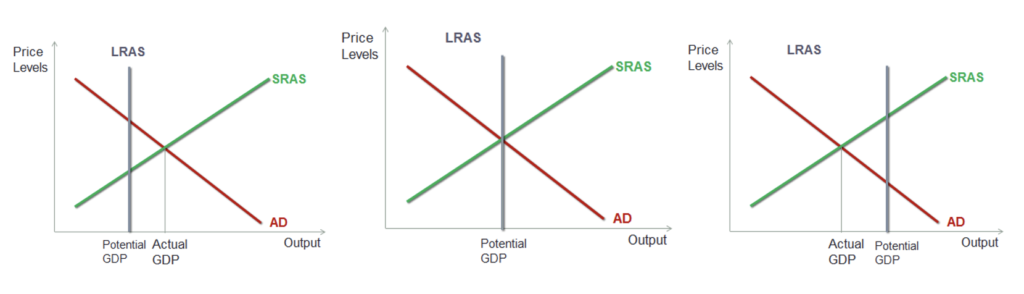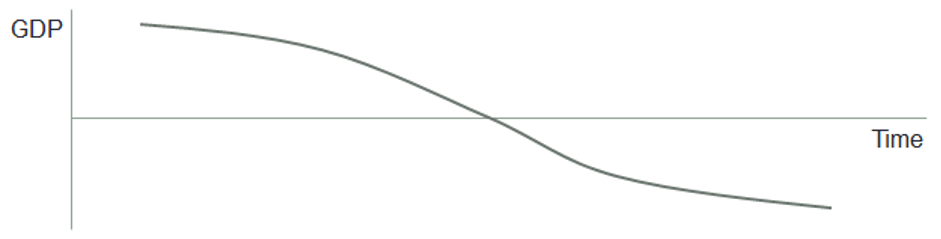If the Aggregate Demand (AD) curve, SRAS and LRAs all intersect at one point, then the economy is in long run and short run equilibrium. However, there could be situations where the economy is in short-run equilibrium but not in long-run equilibrium. Consider the following three figures:The output at the long run equilibrium is the potential GDP while the output at the short run equilibrium is the actual GDP.

In the first graph, the actual GDP is above the potential GDP, i.e., the full employment GDP. This is called expansion or inflationary gap.

In the second graph, the AD, SRAS as well as LRAS intersect at the same point. The economy is in short-run as well as long-run equilibrium and real GDP is equal to potential GDP.

In the third graph, the real GDP is less than potential GDP. The above three graphs related to different phases in business cycle, as shown below: